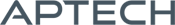Nonlinear Equations MT

Nonlinear Equations MT

Availability: Out of stock

The Nonlinear Equations MT applications module (NLSYS) solves systems of nonlinear equations where there are as many equations as unknowns.
Overview

Nonlinear Equations MT

The Nonlinear Equations MT applications module (NLSYS) solves systems of nonlinear equations where there are as many equations as unknowns. This application is thread-safe and takes advantage of structures found in later versions of GAUSS.

The functions must be continuous and differentiable. You may provide a function for calculating the Jacobian, if desired. Otherwise NLSYS will compute the Jacobian numerically. NLSYS solves a system of nonlinear equations using a quasi-Newton algorithm with Broyden's secant updated method. You an choose either a line search algorithm or a hookstep method.

Platform: Windows, Mac and Linux.

Requirements: GAUSS/GAUSS Engine/GAUSS Light v8.0 or higher.

Product Inquiry

1 + 4 = enter the result ex:(3 + 2 = 5)

﻿